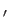Next: Representing Graphs as Bit Up: Preliminaries Previous: Evasive Functions:   Contents

#### Graph Properties:

A graph property is a set of graphs closed under graph isomorphism. If a graph property holds for some graph G, it must hold for all graphs Gisomorphic to G. For example: Is there an edge from vertex 1 to vertex 2?'' is not a graph property, but Does the graph have one edge?'' is. Some graph properties are symmetric, such as Is the graph complete?'' Others exhibit symmetry in a different sense than that of Definition 2.5.1, such as Does the graph contain a vertex of degree 5?''

A non-trivial graph property is one that is false for some graph, and true for some graph. If adding an edge to a graph can not make a property fail the graph property is called monotone. Examples of well-known monotone graph properties are whether a graph is connected, acyclic, non-bipartite, complete, non-planar, or non-k-colorable. Nonmonotone graph properties include whether the graph is a tree and k-regularity.

Conjecture 3.1.1 (Aanderaa-Karp-Rosenberg)   All non-trivial monotone graph properties are evasive in the classical deterministic setting.

Conjecture 3.1.1 is known to be true for graphs whose number of vertices is a prime power .

The conjecture makes monotone graph properties an appealing target for study in the quantum oracle model. We attained asymptotically tight lower bounds for evasive symmetric functions in Chapter 2. In non-trivial monotone graph properties we have a class of functions, conjectured to be evasive, that exhibit a kind of symmetry due to their invariance under relabeling of vertices. We hope that Ambainis' Theorem can provide us with asymptotically tight lower bounds for non-trivial monotone graph properties as they did for nonconstant symmetric functions.Next: Representing Graphs as Bit Up: Preliminaries Previous: Evasive Functions:   Contents
Matthew Hayward Lower Query Bounds in the Quantum Oracle Model GitHub Repository